• 理想低通滤波器容许低频信号通过, 但减弱(或减少)频率高于截止频率的信号的通过。对于不同滤波器而言,每个频率的信号的减弱程度不同。当使用在音频应用时,它有时被称为高频剪切滤波器, 或高音消除滤波器。
• 理想低通滤波器 (ILPF)：简单地切断所有距离变换原点指定距离matlab
• 低通滤波器  1.理想的低通滤波器  其中，D0表示通带的半径。D(u,v)的计算方式也就是两点间的距离，很简单就能...由于理想低通滤波器的过度特性过于急峻，所以会产生了振铃现象。
低通滤波器

1.理想的低通滤波器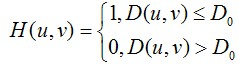其中，D0表示通带的半径。D(u,v)的计算方式也就是两点间的距离，很简单就能得到。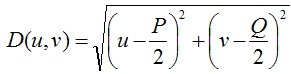使用低通滤波器所得到的结果如下所示。低通滤波器滤除了高频成分，所以使得图像模糊。由于理想低通滤波器的过度特性过于急峻，所以会产生了振铃现象。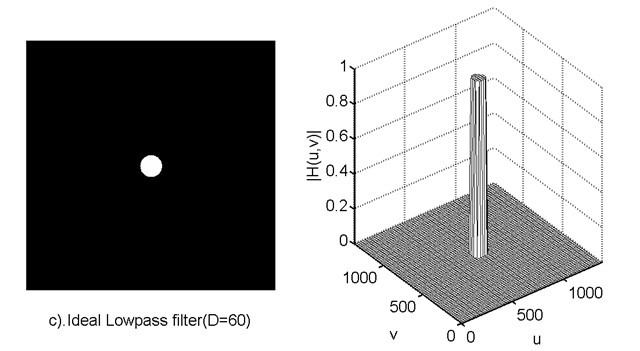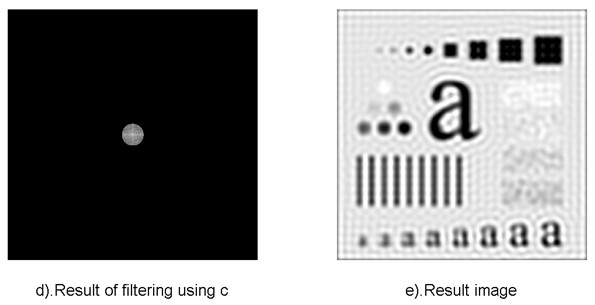2.巴特沃斯低通滤波器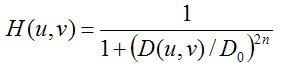同样的，D0表示通带的半径，n表示的是巴特沃斯滤波器的次数。随着次数的增加，振铃现象会越来越明显。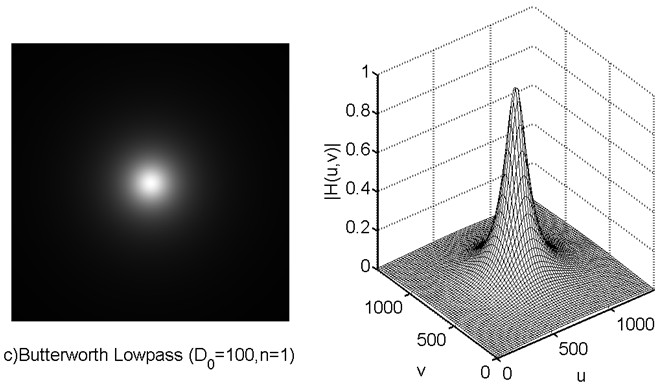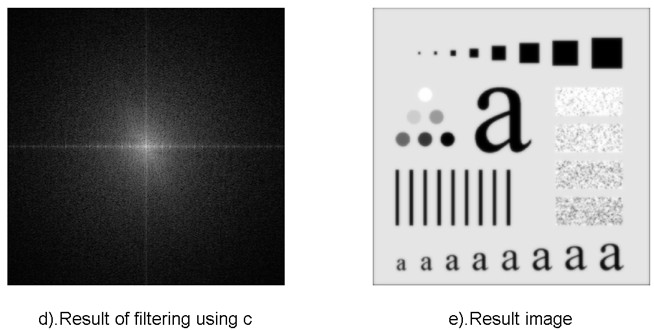3.高斯低通滤波器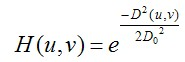D0表示通带的半径。高斯滤波器的过度特性非常平坦，因此是不会产生振铃现象的。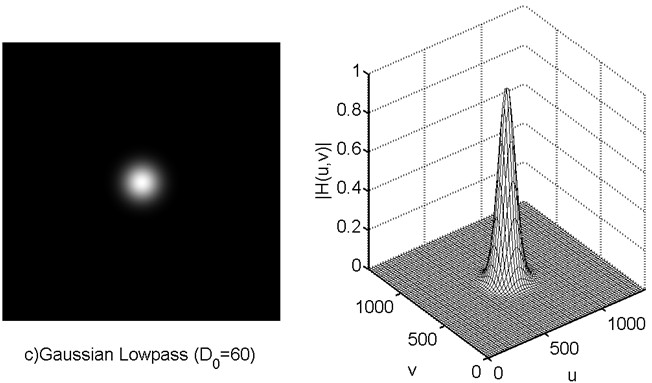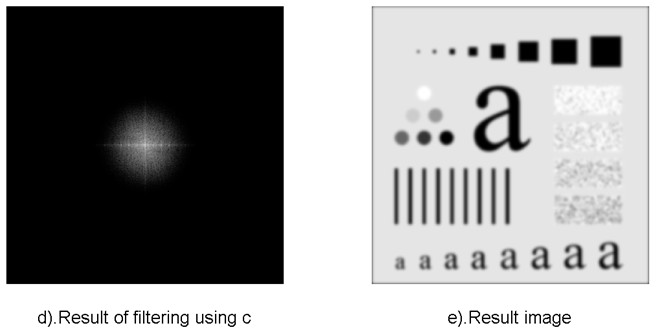展开全文• 首先看个图了解下什么是理想低通滤波器公式和图是转自Rolin的专栏 低通滤波器   1.理想的低通滤波器  其中，D0表示通带的半径。D(u,v)的计算方式也就是两点间的距离，很简单就能得到。  使用低...

首先看个图了解下什么是理想低通滤波器公式和图是转自Rolin的专栏

低通滤波器

1.理想的低通滤波器其中，D0表示通带的半径。D(u,v)的计算方式也就是两点间的距离，很简单就能得到。使用低通滤波器所得到的结果如下所示。低通滤波器滤除了高频成分，所以使得图像模糊。由于理想低通滤波器的过度特性过于急峻，所以会产生了振铃现象。2.巴特沃斯低通滤波器同样的，D0表示通带的半径，n表示的是巴特沃斯滤波器的次数。随着次数的增加，振铃现象会越来越明显。void ideal_Low_Pass_Filter(Mat src){
Mat img;
cvtColor(src, img, CV_BGR2GRAY);
imshow("img",img);
//调整图像加速傅里叶变换
int M = getOptimalDFTSize(img.rows);
int N = getOptimalDFTSize(img.cols);
copyMakeBorder(img, padded, 0, M - img.rows, 0, N - img.cols, BORDER_CONSTANT, Scalar::all(0));
//记录傅里叶变换的实部和虚部
Mat complexImg;
merge(planes, 2, complexImg);
//进行傅里叶变换
dft(complexImg, complexImg);
//获取图像
Mat mag = complexImg;
mag = mag(Rect(0, 0, mag.cols & -2, mag.rows & -2));//这里为什么&上-2具体查看opencv文档
//其实是为了把行和列变成偶数 -2的二进制是11111111.......10 最后一位是0
//获取中心点坐标
int cx = mag.cols / 2;
int cy = mag.rows / 2;
//调整频域
Mat tmp;
Mat q0(mag, Rect(0, 0, cx, cy));
Mat q1(mag, Rect(cx, 0, cx, cy));
Mat q2(mag, Rect(0, cy, cx, cy));
Mat q3(mag, Rect(cx, cy, cx, cy));

q0.copyTo(tmp);
q3.copyTo(q0);
tmp.copyTo(q3);

q1.copyTo(tmp);
q2.copyTo(q1);
tmp.copyTo(q2);
//Do为自己设定的阀值具体看公式
double D0 = 60;
//处理按公式保留中心部分
for (int y = 0; y < mag.rows; y++){
double* data = mag.ptr<double>(y);
for (int x = 0; x < mag.cols; x++){
double d = sqrt(pow((y - cy),2) + pow((x - cx),2));
if (d <= D0){

}
else{
data[x] = 0;
}
}
}
//再调整频域
q0.copyTo(tmp);
q3.copyTo(q0);
tmp.copyTo(q3);
q1.copyTo(tmp);
q2.copyTo(q1);
tmp.copyTo(q2);
//逆变换
Mat invDFT, invDFTcvt;
idft(mag, invDFT, DFT_SCALE | DFT_REAL_OUTPUT); // Applying IDFT
invDFT.convertTo(invDFTcvt, CV_8U);
imshow("理想低通滤波器", invDFTcvt);
}

void Butterworth_Low_Paass_Filter(Mat src){
int n = 1;//表示巴特沃斯滤波器的次数
//H = 1 / (1+(D/D0)^2n)
Mat img;
cvtColor(src, img, CV_BGR2GRAY);
imshow("img", img);
//调整图像加速傅里叶变换
int M = getOptimalDFTSize(img.rows);
int N = getOptimalDFTSize(img.cols);
copyMakeBorder(img, padded, 0, M - img.rows, 0, N - img.cols, BORDER_CONSTANT, Scalar::all(0));

Mat complexImg;
merge(planes, 2, complexImg);

dft(complexImg, complexImg);

Mat mag = complexImg;
mag = mag(Rect(0, 0, mag.cols & -2, mag.rows & -2));

int cx = mag.cols / 2;
int cy = mag.rows / 2;

Mat tmp;
Mat q0(mag, Rect(0, 0, cx, cy));
Mat q1(mag, Rect(cx, 0, cx, cy));
Mat q2(mag, Rect(0, cy, cx, cy));
Mat q3(mag, Rect(cx, cy, cx, cy));

q0.copyTo(tmp);
q3.copyTo(q0);
tmp.copyTo(q3);

q1.copyTo(tmp);
q2.copyTo(q1);
tmp.copyTo(q2);

double D0 = 100;

for (int y = 0; y < mag.rows; y++){
double* data = mag.ptr<double>(y);
for (int x = 0; x < mag.cols; x++){
//cout << data[x] << endl;
double d = sqrt(pow((y - cy), 2) + pow((x - cx), 2));
//cout << d << endl;
double h = 1.0 / (1 + pow(d / D0, 2 * n));
if (h <= 0.5){
data[x] = 0;
}
else{
//data[x] = data[x]*0.5;
//cout << h << endl;
}

//cout << data[x] << endl;
}
}
q0.copyTo(tmp);
q3.copyTo(q0);
tmp.copyTo(q3);
q1.copyTo(tmp);
q2.copyTo(q1);
tmp.copyTo(q2);
//逆变换
Mat invDFT, invDFTcvt;
idft(complexImg, invDFT, DFT_SCALE | DFT_REAL_OUTPUT); // Applying IDFT
invDFT.convertTo(invDFTcvt, CV_8U);
imshow("巴特沃斯低通滤波器", invDFTcvt);
}




展开全文opencv
• 精选文档 DSP设计滤波器报告 一低通滤波器的设计 一实验目的掌握 IIR 数字低通滤波器的设计方法 二实验原理 1滤波器的分类 滤波器分两大类经典滤波器和现代滤波器 经典滤波器是假定输入信号 x( n) 中的有用成分和...
• 理想低通滤波器设计,MATLAB代码，实现低通滤波
• 目的-本文的目的是提出一种具有非理想低通滤波器的调制宽带转换器（MWC）的模型校准技术。 在不对系统架构进行任何更改的情况下，以适度的过采样为代价，校准后的系统可以充当具有理想低通滤波器的系统。 设计/方法/...
• 本课件重点讲述了理想低通滤波器的概念和基本知识，很详细的介绍了理想低通滤波器方面的基本知识。
•  1、理想低通滤波器  2、巴特沃思低通滤波器  3、高斯低通滤波器   √ 边缘和噪声等尖锐变化处于傅里叶变换的高 频部分 √ 平滑可以通过衰减高频成分的范围来实现 √ 理想低通滤波器：尖锐 √ 巴特沃思低...
频率域平滑滤波器有
1、理想低通滤波器
2、巴特沃思低通滤波器
3、高斯低通滤波器

√ 边缘和噪声等尖锐变化处于傅里叶变换的高 频部分
√   平滑可以通过衰减高频成分的范围来实现
√   理想低通滤波器：尖锐
√   巴特沃思低通滤波器：处于理想和高斯滤波 器之间
√   高斯低通滤波器：平滑

l  理想低通滤波器
√    截断傅里叶变换中的所有高频成分，这些高 频成分处于指定距离D0之外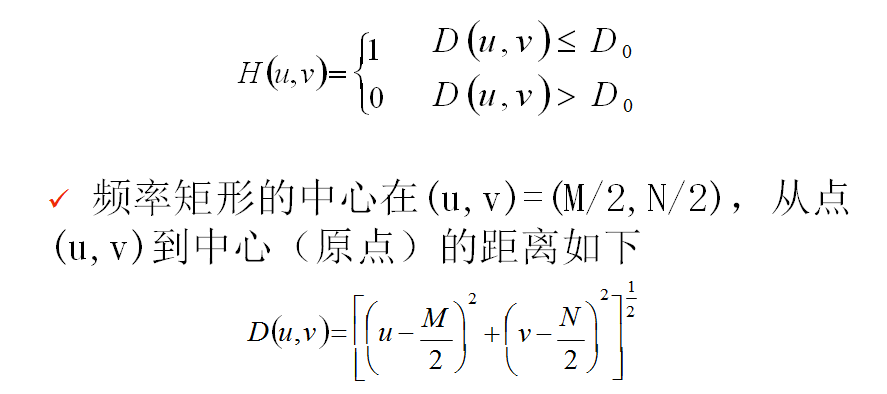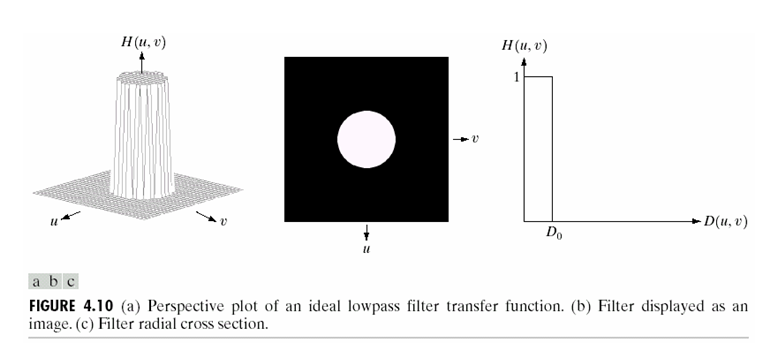说明：在半径为D0的圆内，所有频率没有衰减地通过滤 波器，而在此半径的圆之外的所有频率完全被衰减掉

原图像：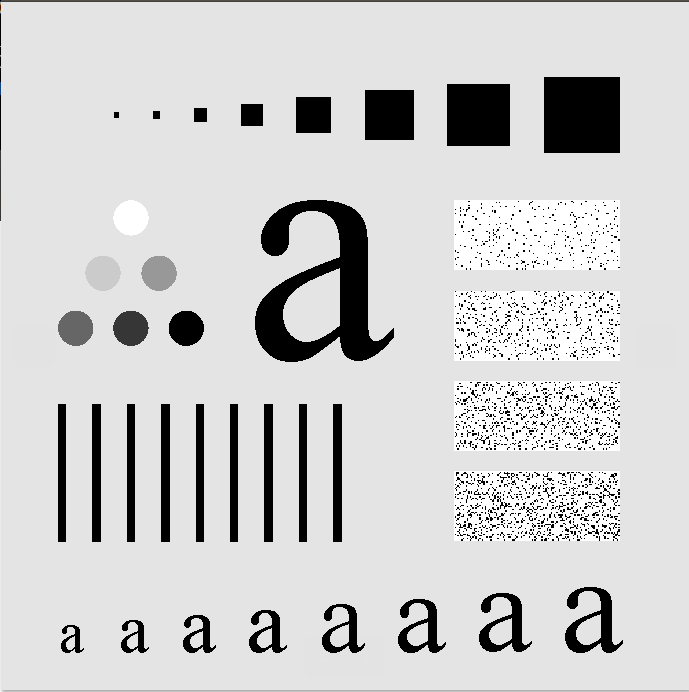截止频率的半径值=10：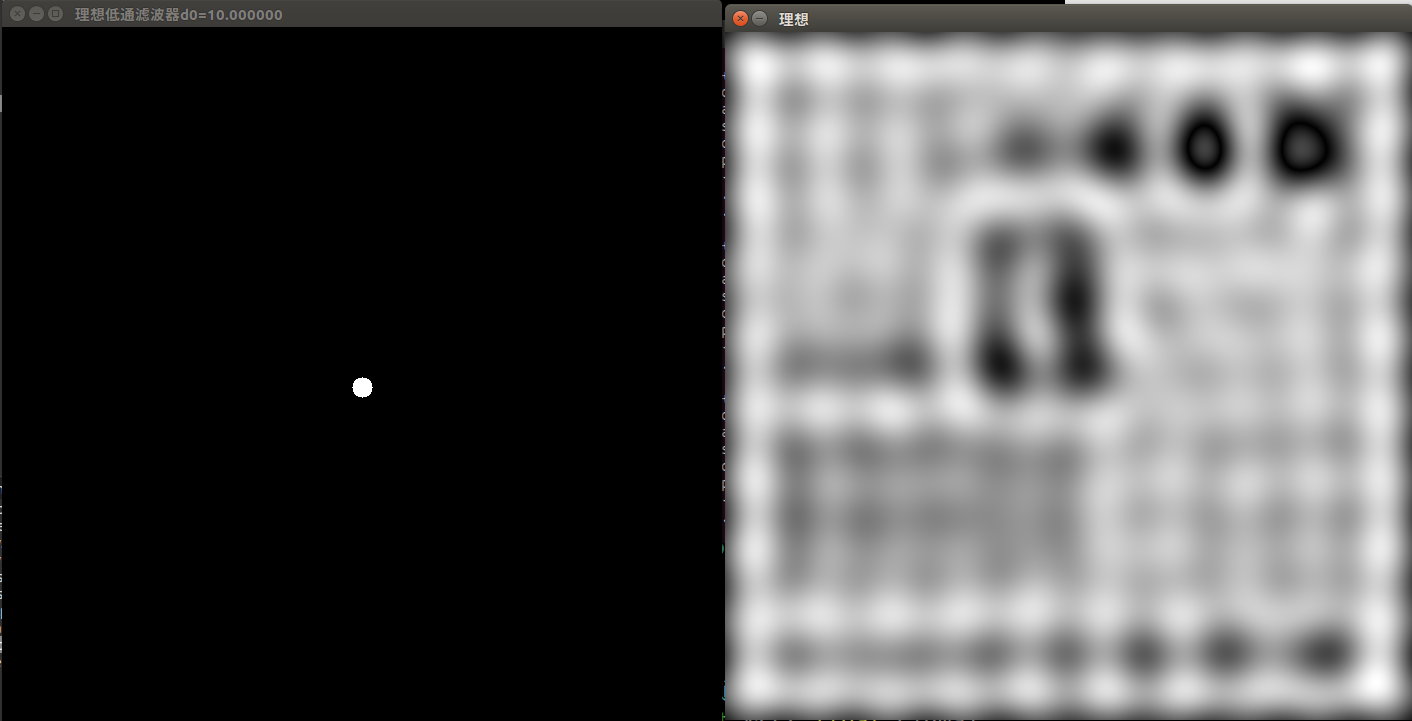截止频率的半径值=50：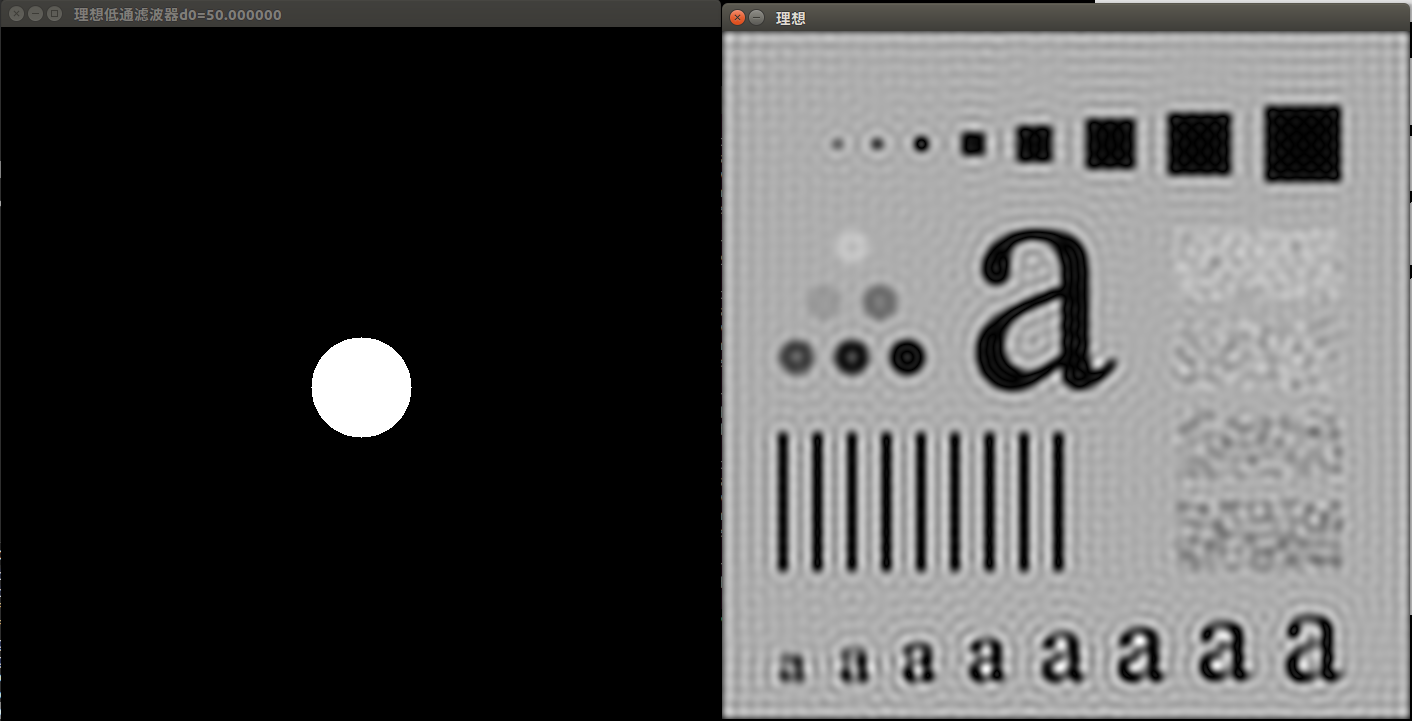截止频率的半径值=160：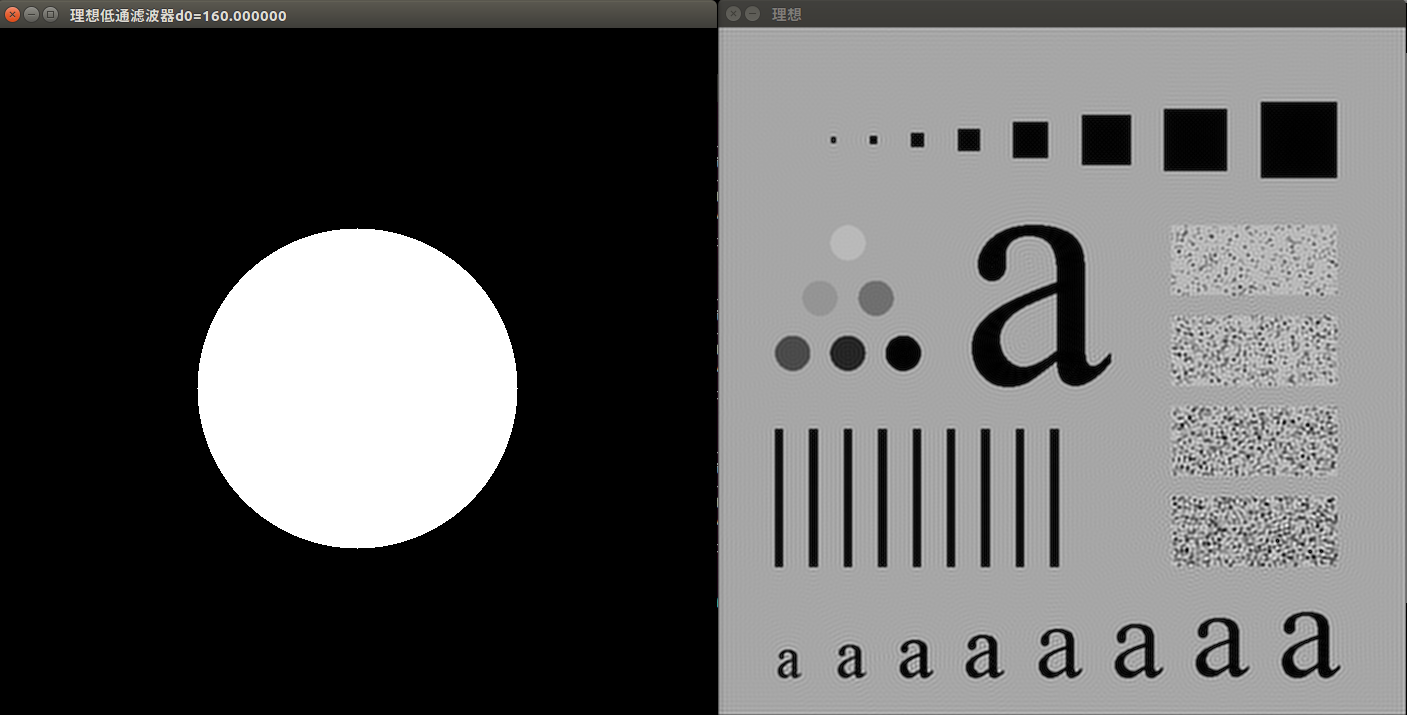代码实现：
#include<opencv2/opencv.hpp> #include<iostream>
using namespace std; using namespace cv;
cv::Mat ideal_Low_Pass_Filter(Mat &src, float sigma); Mat ideal_lbrf_kernel(Mat &scr,float sigma); Mat freqfilt(Mat &scr,Mat &blur);
int main( int argc, char *argv[]) {     const char* filename = argc >=2 ? argv : "../data/lena.jpg";
cv::Mat ideal = ideal_Low_Pass_Filter(input, 160);     ideal = ideal(cv::Rect(0,0, input.cols, input.rows));     imshow("理想", ideal);     waitKey();     return 0; }
//*****************理想低通滤波器*********************** Mat ideal_lbrf_kernel(Mat &scr,float sigma) {     Mat ideal_low_pass(scr.size(),CV_32FC1); //，CV_32FC1     float d0=sigma;//半径D0越小，模糊越大；半径D0越大，模糊越小     for(int i=0;i<scr.rows ; i++ ){         for(int j=0; j<scr.cols ; j++ ){             double d = sqrt(pow((i - scr.rows/2),2) + pow((j - scr.cols/2),2));//分子,计算pow必须为float型             if (d <= d0){                 ideal_low_pass.at<float>(i,j)=1;             }             else{               ideal_low_pass.at<float>(i,j)=0;             }         }     }     string name = "理想低通滤波器d0=" + std::to_string(sigma);     imshow(name, ideal_low_pass);     return ideal_low_pass; }
cv::Mat ideal_Low_Pass_Filter(Mat &src, float sigma) {     int M = getOptimalDFTSize(src.rows);     int N = getOptimalDFTSize(src.cols);     Mat padded;                 //调整图像加速傅里叶变换     copyMakeBorder(src, padded, 0, M - src.rows, 0, N - src.cols, BORDER_CONSTANT, Scalar::all(0));     padded.convertTo(padded,CV_32FC1); //将图像转换为flaot型
Mat ideal_kernel=ideal_lbrf_kernel(padded,sigma);//理想低通滤波器     Mat result = freqfilt(padded,ideal_kernel);     return result; } //*****************频率域滤波******************* Mat freqfilt(Mat &scr,Mat &blur) {     //***********************DFT*******************     Mat plane[]={scr, Mat::zeros(scr.size() , CV_32FC1)}; //创建通道，存储dft后的实部与虚部（CV_32F，必须为单通道数）     Mat complexIm;     merge(plane,2,complexIm);//合并通道 （把两个矩阵合并为一个2通道的Mat类容器）     dft(complexIm,complexIm);//进行傅立叶变换，结果保存在自身
//***************中心化********************     split(complexIm,plane);//分离通道（数组分离） //    plane = plane(Rect(0, 0, plane.cols & -2, plane.rows & -2));//这里为什么&上-2具体查看opencv文档 //    //其实是为了把行和列变成偶数 -2的二进制是11111111.......10 最后一位是0     int cx=plane.cols/2;int cy=plane.rows/2;//以下的操作是移动图像  (零频移到中心)     Mat part1_r(plane,Rect(0,0,cx,cy));  //元素坐标表示为(cx,cy)     Mat part2_r(plane,Rect(cx,0,cx,cy));     Mat part3_r(plane,Rect(0,cy,cx,cy));     Mat part4_r(plane,Rect(cx,cy,cx,cy));
Mat temp;     part1_r.copyTo(temp);  //左上与右下交换位置(实部)     part4_r.copyTo(part1_r);     temp.copyTo(part4_r);
part2_r.copyTo(temp);  //右上与左下交换位置(实部)     part3_r.copyTo(part2_r);     temp.copyTo(part3_r);
Mat part1_i(plane,Rect(0,0,cx,cy));  //元素坐标(cx,cy)     Mat part2_i(plane,Rect(cx,0,cx,cy));     Mat part3_i(plane,Rect(0,cy,cx,cy));     Mat part4_i(plane,Rect(cx,cy,cx,cy));
part1_i.copyTo(temp);  //左上与右下交换位置(虚部)     part4_i.copyTo(part1_i);     temp.copyTo(part4_i);
part2_i.copyTo(temp);  //右上与左下交换位置(虚部)     part3_i.copyTo(part2_i);     temp.copyTo(part3_i);
//*****************滤波器函数与DFT结果的乘积****************     Mat blur_r,blur_i,BLUR;     multiply(plane, blur, blur_r); //滤波（实部与滤波器模板对应元素相乘）     multiply(plane, blur,blur_i);//滤波（虚部与滤波器模板对应元素相乘）     Mat plane1[]={blur_r, blur_i};     merge(plane1,2,BLUR);//实部与虚部合并
//*********************得到原图频谱图***********************************     magnitude(plane,plane,plane);//获取幅度图像，0通道为实部通道，1为虚部，因为二维傅立叶变换结果是复数     plane+=Scalar::all(1);  //傅立叶变换后的图片不好分析，进行对数处理，结果比较好看     log(plane,plane);    // float型的灰度空间为[0，1])     normalize(plane,plane,1,0,CV_MINMAX);  //归一化便于显示 //    imshow("原图像频谱图",plane);
idft( BLUR, BLUR);    //idft结果也为复数     split(BLUR,plane);//分离通道，主要获取通道     magnitude(plane,plane,plane);  //求幅值(模)     normalize(plane,plane,1,0,CV_MINMAX);  //归一化便于显示     return plane;//返回参数 }


展开全文• 数字图像处理，评语滤波，理想低通滤波器，高斯滤波器，巴特沃斯滤波器数字图像处理
• 探究并给出了理想低通滤波器的码元速率的条件
奈奎斯特准则与带限信道可行的码元速率探究（篇三）:理想低通滤波器的可行码元速率探究
写在前：
本篇是《奈奎斯特准则与带限信道可行的码元速率探究》的篇三，我的个人探究部分。主要探究并给出了理想低通滤波器的码元速率的条件。本系列文章的篇一：什么是码间串扰介绍了码间串扰的基本概念和原理。篇二：基带传输系统的奈奎斯特准则与奈奎斯特速率介绍了基带传输系统中的码间串扰的解决、奈奎斯特准则与奈奎斯特速率。
首先我考虑了不满足的速率，自然想到的就是

R

s

R_s

与

2

W

2W

之间存在“乖张古怪”的无理数倍乘因子时一定不满足无码间干扰，因为自我感觉无理数都满足了的话有理数就更能满足了，也自己尝试着用无理数

π

\pi

做码率画图观察证实。可这毕竟是无理数啊，也画不准，画着画着就凌乱了。
所以，换个方式吧，并且严谨一点，设标号求一般值吧，试试。 来看图1。图

1

R

s

⩽

2

W

的

情

况

图1\ R_s\leqslant2W的情况

我们已经知道码率最高为

R

s

max

⁡

=

2

W

R_{s\ \max}=2W

，为了探究

R

s

⩽

2

W

R_s\leqslant2W

的情况，现在令

R

s

=

2

W

/

a

R_s=2W /a

，其中

a

⩾

1

a\geqslant 1

（这里，我们试图同时探究和合并

R

s

=

2

W

R_s=2W

和

R

s

&lt;

2

W

R_s&lt;2W

情况）。根据

∑

k

=

−

∞

∞

H

(

f

−

k

⋅

R

s

)

\sum_{k=-\infty}^\infty H(f-k\cdot R_s)

的周期性，只需观察它在

(

−

R

s

/

2

，

R

s

/

2

)

(-R_s/2，R_s/2)

上是否为常数，而

−

W

⩽

−

R

s

/

2

&lt;

R

s

/

2

⩽

W

-W\leqslant -R_s/2&lt;R_s/2\leqslant W

，为了后面的方便我们不妨扩大一点范围讨论其在

(

−

W

，

W

)

(-W，W)

上是否为常数。
（1）首先，只考虑

k

⩾

0

k\geqslant 0

的情形：
在区间

(

−

W

,

−

W

+

R

s

)

(-W,-W+R_s)

上有且只有

k

=

0

k=0

的贡献；
在区间

(

−

W

+

R

s

,

−

W

+

2

R

s

)

(-W+R_s,-W+2R_s)

上有且只有

k

=

0

,

1

k=0,1

的贡献；
在区间

(

−

W

+

2

R

s

,

−

W

+

3

R

s

)

(-W+2R_s,-W+3R_s)

上有且只有

k

=

0

,

1

,

2

k=0,1,2

的贡献；
……
在区间

(

−

W

+

m

⋅

R

s

,

−

W

+

(

m

+

1

)

⋅

R

s

)

(-W+m\cdot R_s,-W+(m+1)\cdot R_s)

上有且只有

k

=

0

,

1

,

2

,

⋯
&ThinSpace;

,

m

k=0,1,2,\cdots ,m

的贡献，其中

W

∈

(

−

W

+

m

⋅

R

s

,

−

W

+

(

m

+

1

)

⋅

R

s

)

W\in (-W+m\cdot R_s,-W+(m+1)\cdot R_s)

，得

m

⩽

a

−

1

m\leqslant a-1

；
贡献的份数随着子区间自左向右逐渐递增。
（2）再来考虑

k

⩽

0

k\leqslant 0

的情形：
在区间

(

W

−

R

s

,

W

)

(W-R_s,W)

上有且只有

k

=

0

k=0

的贡献；
在区间

(

W

−

2

R

s

,

W

−

R

s

)

(W-2R_s,W-R_s)

上有且只有

k

=

0

,

−

1

k=0,-1

的贡献；
在区间

(

W

−

3

R

s

,

W

−

2

R

s

)

(W-3R_s,W-2R_s)

上有且只有

k

=

0

,

−

1

,

−

2

k=0,-1,-2

的贡献；
……
在区间

(

W

−

(

m

+

1

)

⋅

R

s

,

W

−

m

⋅

R

s

)

(W-(m+1)\cdot R_s,W-m\cdot R_s)

上有且只有

k

=

0

,

−

1

,

−

2

,

⋯
&ThinSpace;

,

−

m

k=0,-1,-2,\cdots ,-m

的贡献，其中满足

W

∈

(

W

−

(

m

+

1

)

⋅

R

s

,

W

−

m

⋅

R

s

)

W\in (W-(m+1)\cdot R_s,W-m\cdot R_s)

，依旧得

m

⩽

a

−

1

m\leqslant a-1

；
贡献的份数随着子区间自右向左逐渐递增。
现在综合考虑以上（1）、（2）两种贡献，根据自左向右（

k

⩾

0

k\geqslant 0

）和自右向左（

k

⩽

0

k\leqslant 0

）的贡献的对称性相加，在区间

(

−

W

，

W

)

(-W，W)

上

∑

k

=

−

∞

∞

H

(

f

−

k

⋅

R

s

)

\sum_{k=-\infty}^\infty H(f-k\cdot R_s)

为常数的充要条件转化为：

y

=

{

n

=

a

−

1

(

1.0

)

是

最

左

或

最

右

区

间

的

条

件

−

W

=

W

−

(

n

+

1

)

⋅

R

s

(

1.1

)

最

左

区

间

的

左

边

界

条

件

−

W

+

R

s

=

W

−

n

R

s

(

1.2

)

最

左

区

间

的

右

边

界

条

件

−

W

+

n

R

s

=

W

−

R

s

(

1.3

)

最

右

区

间

的

左

边

界

条

件

−

W

+

(

n

+

1

)

⋅

R

s

=

W

(

1.4

)

最

右

区

间

的

左

边

界

条

件

y = \left\{ \begin{array}{ll} n=a-1\ \ \ \ \ \ \ \ \ \ \ \ \ \ \ \ \ \ \ \ \ \ \ \ \ \ \ \ \ (1.0)是最左或最右区间的条件\\ -W=W-(n+1)\cdot R_s\ \ \ \ (1.1)最左区间的左边界条件\\ -W+R_s=W-nR_s\ \ \ \ \ \ \ (1.2)最左区间的右边界条件\\ -W+nR_s=W-R_s\ \ \ \ \ \ \ (1.3)最右区间的左边界条件\\ -W+(n+1)\cdot R_s=W\ \ \ \ (1.4)最右区间的左边界条件\\ \end{array} \right.

可以得出这五个式子均等价于：

a

=

n

+

1

a=n+1

其中，

R

s

=

R

s

max

⁡

/

a

=

2

W

/

a

R_s=R_{s \max}/a=2W/a

，

n

n

的取值范围是

{

0

,

1

,

2

,

3

,

⋯
&ThinSpace;

}

\{0,1,2,3,\cdots \}

，所以相应的

a

a

的取值范围是

{

1

,

2

,

3

,

4

,

⋯
&ThinSpace;

}

\{1,2,3,4,\cdots \}

。至此我们可以得出结论：
理想低通信道（理想LPF）的可行码率为最高码率除以一个正整数，其中最高码率为

R

s

max

⁡

/

a

=

2

W

/

a

R_{s \max}/a=2W/a

。
至此，我们得出了理想低通信道的可行码率，其余的理想带限信道可类比。
编后：
这个很简单简洁的知识和结论得到了我的老师的肯定，问老师，为什么书上没提过这个小结论呢，您之间也不知道具体条件。老师说可能是因为主要关注和应用的是最高码率，其他可行的低的码率浪费信道资源（效率低），大家就不会来考虑了；而且，我们谈论的是理想信道，实际并非这般理想。   所以，我这个可能是脱离实际的无效的理论探究，但是很享受其中的过程和思路。有时候常识和直觉不一定靠谱，通过严谨的甚至会是很简单的数学性的探究可以给我们答案和肯定。常识和直觉可能是一种知识一种智慧，也可能是我们认识真理的阻碍。
展开全文奈奎斯特准则 码元速率 带限信道
• 低通滤波器概念有许多不同的形式，其中包括电子线路（如音频设备中使用的hiss 滤波器）、平滑数据的数字算法、音障（acousc barriers）、图像模糊处理等等，这两个工具都通过剔除短期波动、保留长期发展趋势提供了...
• 低通滤波器概念有许多不同的形式，其中包括电子线路(如音频设备中使用的hiss 滤波器)、平滑数据的数字算法、音障(acousTIc barriers)、图像模糊处理等等，这两个工具都通过剔除短期波动、保留长期发展趋势提供了信号...
• 一、简介我们知道，在一幅图像中，其低频成分对应者图像变化缓慢的部分，对应着图像大致的相貌和轮廓。而其高频成分则对应着图像变化剧烈的部分，对应着图像的细节（图像的噪声也属于高频成分）。...理想低通滤波器...matlab编程
• # 理想低通滤波器 # 导入相关库 from skimage import data, color import numpy as np import matplotlib.pyplot as plt """ 中文显示工具函数 """ def set_ch(): from pylab import mpl mpl.rcParams['font....python 深度学习
• 典型低通滤波器；%0.1 Hd(r>0.1) = 0; Y=fft2(double(I)); Y=fftshift(Y); Ya=Y.*Hd;¨ Ya=ifftshift(Ya); Ia01=ifft2(Ya); %0.2 Hd(r>0.2) = 0; ÷ Y=fft2(double(I)); Y=fftshift(Y); Ya=Y.*Hd; ...
• 低通滤波器    1.理想的低通滤波器 ...D(u,v)的计算方式也就是两点间的距离，很简单就能得到。...由于理想低通滤波器的过度特性过于急峻，所以会产生了振铃现象。      2.巴特沃斯低通滤波器
• 毕业论文设计 论文题目 基于 MATLAB 的高阶低通滤波器的设计与仿真 学生姓名 学 号 0908030222 所在院系 电气信息工程学院 专业名称 电子信息工程 届 次 2013 届 指导教师 l 目 录 前言 2 1 滤波器 2 1.1 滤波器的...
• 1、实验要求：对如下图像使用半径分别为5, 15, 30的理想低通滤波器进行处理，并分别显示结果图像。 2、实验过程：代码如下 %频率域滤波  clc;close all;  img=imread('1.tif');  img=im2double(img);    Fimg...matlab
• 理想低通滤波器 f = imread('Fig0441.tif'); f = im2double(f); % 计算填充图像大小 [M,N] = size(f); M2 = 2*M; N2 = 2*N; % 傅里叶变换 F = fftshift(fft2(f,M2,N2)); figure;imshow(mat2gray(log(1+abs(F))))...计算机视觉 matlab 高通滤波器 布特沃斯
• 要使用过滤器： 1）在变量img中创建一张图片2) 调用函数 filter 来创建与图像 'img' 大小相同的过滤器 在滤波器代码中指定截止频率 d0 的值。matlab
•tag
• 低通滤波器概念有许多不同的形式，其中包括电子线路(如音频设备中使用的hiss 滤波器)、平滑数据的数字算法、音障(acoustic barriers)、图像模糊处理等等，这两个工具都通过剔除短期波动、保留长期发展趋势提供了信号......